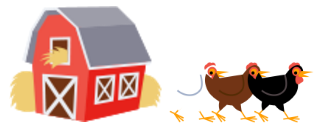Smartick is an advanced online program that teaches kids math and coding in only 15 min. a day
Try it for free!

Dec03

# How to Solve Subtraction Problems

In this post, we are going to learn to analyze, think about, and solve problems that require subtraction. At Smartick, we believe that a student understands and knows math the best when they have the ability to solve problems correctly. Therefore, we are going to analyze 5 different problems that require subtraction to reach a solution.

## Subtraction Problems nº 1:

The book that I am reading has 15 pages. If I have read 7 pages, how many pages are left for me to read?

This is the easiest type of problem that we will find: it is a short statement, that has only 2 data points and they both have the same unit (pages): 15 pages and 7 pages.  Now, we are only left to think about which operation we have to do. For that, we are going to analyze: if the book has 15 pages and I have read 7, am I left with more than or less than 15 pages to read? The solution to this question is that I will be left with less than 15 pages to read;  therefore, the operation that we have to use is SUBTRACTION.

15 – 7 = 8

I have 8 pages left to read.

## Subtraction Problems nº 2:

Calculate how many lights have gone out my Christmas tree if it has 9 lights in total but only 6 shine.

This problem is of a similar difficulty to the problem before, but what makes it more complicated is the indirect question at the beginning of the statement. In order to know the number of lights that have gone out, we are going to analyze the data points pieces that we have: the Christmas tree has 9 lights, but only 6 shine. Therefore, will more than or less than 9 lights have gone out? The answer is that less will have gone out; therefore, we have to SUBTRACT.

9 – 6 = 3

3 lights have gone out.

## Subtraction Problems nº 3:

On Peter’s farm, there are 6 chickens and on John’s, there are 12. How many fewer chickens are there on Peter’s farm than on John’s?In this problem, we have to compare the two data points pieces: Peter has 6 chickens and John has 12 chickens. In order to respond to the question of how many chickens Peter has minus John’s chickens, we have to first analyze: who has fewer chickens? The answer is Peter. Therefore, in order to know how many fewer Peter has than John, I will have to find the difference between the two. And for that, we have to SUBTRACT.

12 – 6 = 6

Peter has 6 chickens less than John.

## Subtraction Problems nº 4:

Valentina is saving up to buy a computer. This month she has not bought any games, and thus, she has saved 20 dollars, just 4 more than last month. How much money did she save last month?

In this problem, the complication comes for from the comparison between the two data points pieces: this month, she has saved 20 dollars, and last month, she saved 4 dollars less. Did she save more or less money last month than this month? The answer is that she saved less: exactly 4 dollars less. Therefore, in order to know the exact amount that she saved, we have to SUBTRACT.

20 – 4 = 16

Last month, she saved 16 dollars.

## Subtraction Problems nº 5:

The parking garage of the hotel where I spent five days of summer vacation with my parents, my uncles, aunts, and cousins had some cars. After 9 more cars entered, I counted 16 cars in total. How many cars were in the parking garage at the beginning?

In this problem, we have 3 data points, but be careful! Not all are important in finding the answer. They ask us for the cars that were in the garage at the beginning; therefore, the data point about the 5 days is not important and we must not consider that in order to solve the problem. The points pieces that matter to us are: 9 cars entered and then there were 16. We are going to think about the number of cars that there were in the beginning: were there more than or less than 16 cars? The answer is there were less. Therefore, the operation that we have to do is SUBTRACTION.

16 – 9 = 7

In the beginning, there were 7 cars.

We hope you enjoyed this post about subtraction problems.

If you want to learn much more elementary math, try Smartick for free!

Learn More: